## Example Questions

← Previous 1 3 4

### Example Question #1 : Extrapolating Exponential Proportions

The potential energy of a spring, in joules, is given by the equation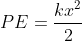where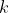is a constant and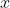is the distance the spring is stretched. If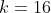and the sprint is stretched to 2 feet and then to 3 feet, how much potential energy, in joules, is gained by the spring from the moment it is stretched 2 feet to the moment it is stretched 3 feet?

32

128

40

72

40

Explanation:

Your first order of business on this problem should be to plug in the value of the constantto simplify your work. When you do so, you have: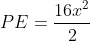which simplifies to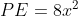.

Now you can plug in the two values ofyou're given. When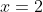the potential energy is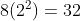.  When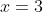the potential energy is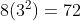. So between 2 and 3 seconds, the spring gains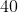joules.

### Example Question #1 : Extrapolating Exponential Proportions

A virus has recently been discovered in the country of Jarvia, and scientists project that the number of people infected by the virus doubles every 3 days. What is the ratio of the number of people who are infected today to the number of people who will be infected 9 days from now?

1:6

1:9

1:8

1:3

1:8

Explanation:

It is important to recognize that this is an exponential rate of increase. If the population doubles every 3 days, then using x as the number of people who would be infected today, you can trace the growth exponentially using:

Today: x people

3 days from now: 2x people

6 days from now: 4x people (2x will have doubled to 4x)

9 days from now: 8x people (4x will have doubled to 8x)

So the correct ratio is 1:8.

### Example Question #3 : Extrapolating Exponential Proportions

On a carousel ride, riders can choose to sit on horses in four zones: zone 1 is closest to the center of the carousel, and zone 4 is the closest to the outer edge. The rate of speed of each horse is defined by the equation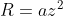, where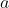is the speed that the ride operator sets for the ride as a whole and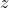represents the zone that the horse is in. If Kenneth is sitting on a horse in Zone 1 and Tamara is sitting on a horse in Zone 4, what is the ratio of the speeds of Tamara to Kenneth during the ride?

4:1

9:1

8:1

16:1

16:1

Explanation:

Sincewill be the same for each rider, you can take this ratio by plugging in their zone numbers.  Tamara's rate would be defined by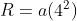or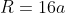and Kenneth's rate would be defined as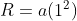or just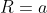. The ratio of their rates, then, is 16:1.

### Example Question #1 : Extrapolating Exponential Proportions

The population of termites in a building can be determined using the equation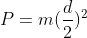, where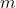is the initial population of the termites and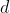is the number of days that termites have been in the building. If the number of termites in a certain building is currently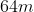, how many days have termites been in that building?

8

16

32

64

16

Explanation:

Whenever you're provided an equation for how to calculate an exponential outcome from an exponential proportion, it's helpful to try to solve the problem algebraically, as often the results are not directly intuitive to try to solve just conceptually.  Here you're given the current population,, and the way that that population can be calculated based on the number of days,. You can then set the population equal toand perform the algebra: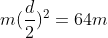You can divide both sides byto simplify: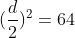And then take the square root of both sides: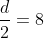Multiply both sides by 2 and you have the answer,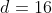### Example Question #5 : Ratios, Proportions, & Percents

A virus has begun spreading through the country of Montrovia. Scientists calculate that the number of infected people doubles every two days. If 64,000 Montrovians have been infected with the virus as of today, how many days has it been since there were 500 people infected with the virus in Montrovia?

12

10

14

7

14

Explanation:

To calculate the answer to this problem, recognize that you're told how often the number of infected people doubles, so your first goal will be to determine how many times the number has doubled from 500 to 64,000. You can start by calculating what number 500 would have to be multiplied by to equal the current 64000: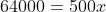So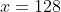You're dealing with an exponential growth proportion in which you know that the virus doubles every two days. So you can express 128 as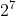, meaning that since there were 500 people infected, the number has doubled 7 times.  Since it takes 2 days for each time the number doubles, you can calculate that it has been 14 days since there were 500 people infected.

### Example Question #5 : Extrapolating Exponential Proportions

Upon discovering a tree virus in British Columbia, scientists created a model to predict the tree population in a certain forest: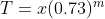, where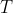represents he number of trees aftermonths since the virus's discovery, andrepresents the original number of trees that existed in the forest when the scientists made the discovery. Assuming that the model is correct, in which of the following years will the number of trees lost be the greatest?

The first year after scientists discovered the virus.

The second year after scientists discovered the virus.

The tree loss will be the same each year.

The third year after the scientists discovered the virus.

The first year after scientists discovered the virus.

Explanation:

Here you are dealing with an exponential rate of decay/loss, as you can see from the fact that the variableis an exponent. What does that mean? That the loss of 27%  (in an exponential loss formula, the portion within parentheses is 1 - %lost; this one "keeps" 73% and subtracts the other 27%) per month compounds each month. Exponential increases and decreases mean that the increase or decrease is taken as a percent of the new value each time: if the forest started with 100 trees it would have lost 27 in the first year (27% of the original 100), and then the next year the 27% decrease would be taken from the new total of 73. Since the "new total" shrinks from year to year, the total number of trees lost will shrink, too, as it is a function of the number of trees to start that year. So here the answer is "the first year" as that is when the starting total will be greatest.

### Example Question #2 : Ratios, Proportions, & Percents

A mobile app video game has gone viral, with its number of users tripling each day for the past several weeks. What is the ratio of its number of users today to its number of users exactly one week ago?

21:1

2187:1

27:1

243:1

2187:1

Explanation:

If the number of users has tripled each day, that reflects an exponential increase. If there were 1 user on day one, then on day two it would be 3 users, then on day three there would be 3x3=9 users, then on day four there would be 9x3=27 and so on. You can account for this by saying that the number of users will be equal to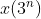, whereis the original number of users and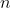is the number of days since the first day. Since you're looking for the number of users 7 days after the first day, you would calculate that as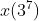. Since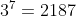, you'd haveusers on day one and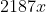users one week later, for a ratio of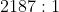.

(And note: this ratio will work on any 7-day range of dates, not just from the first day to the 8th day. Whatever your "original" value is will triple each day for 7 days, so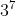times original is what the one-week-later number will be.)

### Example Question #2 : Extrapolating Exponential Proportions

A certain manufacturer of children's toys sells 20% of its starting inventory for the month over the course of each month. If the company manufactures 10,000 units of inventory at the start of the year and does not manufacture any additional units throughout the year, how many toys remain in the manufacturer's inventory at the end of April of that year?

4,096

5,120

2,000

4,000

4,096

Explanation:

Here, we'll want to keep in mind that the base we apply our percent change to changes each month. So, we'll want to take this step by step, and recognize that if the manufacturer sells 20% of its inventory each month, the remaining inventory is 80% of where it started that month. Thus, we can multiply each starting value by 80% as follows

Start of the year: 10,000

End of January: 8,000 (10,000*.8)

End of February: 6,400 (8,000*.8)

End of March: 5,120 (6,400*.8)

End of April: 4,096 (5,120*.8)

So, at the end of April, the manufacturer has 4,096 units of inventory. We could also attack this question by translating 80% into a fraction and cancelling factors once we set up the following equation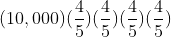to once again arrive at 4096.

### Example Question #8 : Extrapolating Exponential Proportions

A particular variety of water pothos grows by 25% of its current volume each month. If a fish tank contains 64 ounces of pothos at the beginning of March, what volume of pothos will the tank contain at the end of May?

112 ounces

128 ounces

156 ounces

125 ounces

125 ounces

Explanation:

In this example, we'll want to keep in mind that the base we apply our percent change to will be different each month. So, one method of approach would be to take things step by step and apply the calculation of 125% of each months starting volume (a 25% increase) as follows

Beginning March: 64 ounces

End March: 80 ounces (64*1.25)

End April: 100 ounces (80*1.25)

End May: 125 ounces (100*1.25)

So, at the end of May, the tank will contain 125 ounces of the plant.

We could also apply the fractional change to the original amount, using the following expression for the 125%, or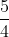change for each of the three months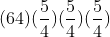Notice, with this setup, our 4*4*4 cancels with the 64 in the numerator, leaving us with 5*5*5, or again, 125 ounces.

### Example Question #1 : Extrapolating Exponential Proportions

A particular ball always bounces back to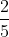of the height of its previous bounce after being dropped. After the first bounce it reaches a height of 625 millimeters. Approximately how high (in inches) will it reach after its fifth bounce?

9.6 millimeters

16 millimeters

24 millimeters

6.4 millimeters

16 millimeters

Explanation:

If the ball begins at a height of 250 millimeters after the first bounce, we can apply the change for each bounce to arrive at the height after the 5th bounce by multiplying each height by.

So, the heights after each bounce are as follows:

After the first bounce - 625

After the second bounce - 250 (625 *)

After the third bounce - 100 (250 *)

After the fourth bounce - 40 (100 *)

After the fifth bounce - 16 (40 *)

Keep in mind, our starting value is already after the first bounce, so we're only applying our multiplier offour times. The common mistake in this question is to assume that because we're looking for the "fifth bounce," we should apply the multiplier five times. Be sure to pay close attention to the wording and, if needed, take things step by step!

← Previous 1 3 4

### All SAT Mathematics Resources